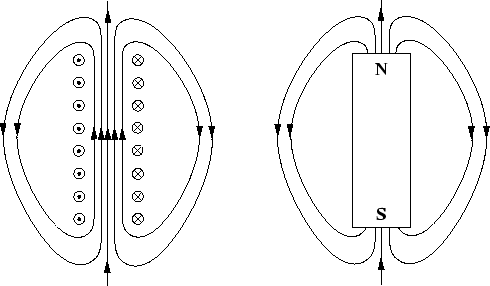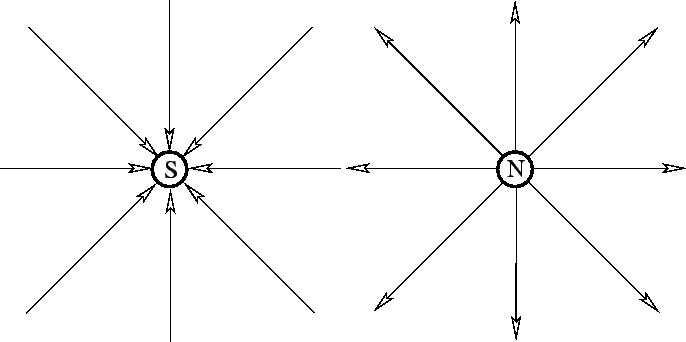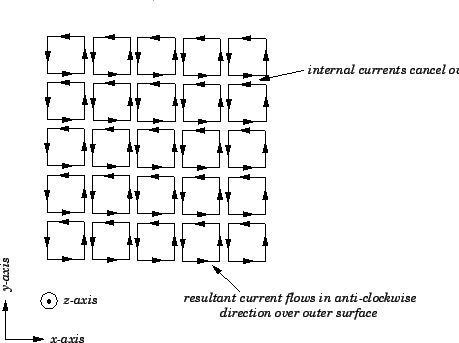Next: Gauss' Law for Magnetic Up: Magnetism Previous: Magnetic Field of a

## Origin of Permanent Magnetism

We now know of two distinct methods of generating a magnetic field. We can either use a permanent magnet, such as a piece of loadstone, or we can run a current around an electric circuit. Are these two methods fundamentally different, or are they somehow related to one another? Let us investigate further.As illustrated in Fig. 28, the external magnetic fields generated by a solenoid and a conventional bar magnet are remarkably similar in appearance. Incidentally, these fields can easily be mapped out using iron filings. The above observation allows us to formulate two alternative hypotheses for the origin of the magnetic field of a bar magnet. The first hypotheses is that the field of a bar magnet is produced by solenoid-like currents which flow around the outside of the magnet, in an anti-clockwise direction as we look along the magnet from its north to its south pole. There is no doubt, by analogy with a solenoid, that such currents would generate the correct sort of field outside the magnet. The second hypothesis is that the field is produced by a positive magnetic monopole located close to the north pole of the magnet, in combination with a negative monopole of equal magnitude located close to the south pole of the magnet. What is a magnetic monopole? Well, it is basically the magnetic equivalent of an electric charge. A positive magnetic monopole is an isolated magnetic north pole. We would expect magnetic field-lines to radiate away from such an object, just as electric field-lines radiate away from a positive electric charge. Likewise, a negative magnetic monopole is an isolated magnetic south pole. We would expect magnetic field-lines to radiate towards such an object, just as electric field-lines radiate towards a negative electric charge. The magnetic field patterns generated by both types of monopole are sketched in Fig. 29. If we place a positive monopole close to the north pole of a bar magnet, and a negative monopole of the same magnitude close to the south pole, then the resultant magnetic field pattern is obtained by superposing the fields generated by the two monopoles individually. As is easily demonstrated, the field generated outside the magnet is indistinguishable from that of a solenoid.We now have two alternative hypotheses to explain the origin of the magnetic field of a bar magnet. What experiment could we perform in order to determine which of these two hypotheses is correct? Well, suppose that we snap our bar magnet in two. What happens according to each hypothesis? If we cut a solenoid in two then we just end up with two shorter solenoids. So, according to our first hypothesis, if we snap a bar magnet in two then we just end up with two smaller bar magnets. However, our second hypothesis predicts that if we snap a bar magnet in two then we end up with two equal and opposite magnetic monopoles. Needless to say, the former prediction is in accordance with experiment, whereas the latter most certainly is not. Indeed, we can break a bar magnetic into as many separate pieces as we like. Each piece will still act like a little bar magnet. No matter how small we make the pieces, we cannot produce a magnetic monopole. In fact, nobody has ever observed a magnetic monopole experimentally, which leads most physicists to conclude that magnetic monopoles do not exist. Thus, we can conclude that the magnetic field of a bar magnet is produced by solenoid-like currents flowing over the surface of the magnet. But, what is the origin of these currents?

In order to answer the last question, let us adopt a somewhat simplistic model of the atomic structure of a bar magnet. Suppose that the north-south axis of the magnet is aligned along the-axis, such that the-coordinate of the magnet's north pole is larger than that of its south pole. Suppose, further, that the atoms which make up the magnet are identical cubes which are packed very closely together. Each atom carries a surface current which circulates in the-plane in an anti-clockwise direction (looking down the-axis). When the atoms are arranged in a uniform lattice, so as to form the magnet, the interior surface currents cancel out, leaving a current which flows only on the outer surface of the magnet. This is illustrated in Fig. 30. Thus, the solenoid-like currents which must flow over the surface of a magnet in order to account for its associated magnetic field are, in fact, just the resultant of currents which circulate in every constituent atom of the magnet. But, what is the origin of these atomic currents?Well, atoms consist of negatively charged electrons in orbit around positively charged nuclei. A moving electric charge constitutes an electric current, so there must be a current associated with every electron in an atom. In most atoms, these currents cancel one another out, so that the atom carries zero net current. However, in the atoms of ferromagnetic materials (i.e., iron, cobalt, and nickel) this cancellation is not complete, so these atoms do carry a net current. Usually, the atomic currents are all jumbled up (i.e., they are not aligned in any particular plane) so that they average to zero on a macroscopic scale. However, if a ferromagnetic material is placed in a strong magnetic field then the currents circulating in each atom become aligned such that they flow predominately in the plane perpendicular to the field. In this situation, the currents can combine together to form a macroscopic magnetic field which reinforces the alignment field. In some ferromagnetic materials, the atomic currents remain aligned after the alignment field is switched off, so the macroscopic field generated by these currents also remains. We call such materials permanent magnets.

In conclusion, all magnetic fields encountered in nature are generated by circulating currents. There is no fundamental difference between the fields generated by permanent magnets and those generated by currents flowing around conventional electric circuits. In the former, case the currents which generate the fields circulate on the atomic scale, whereas, in the latter case, the currents circulate on a macroscopic scale (i.e., the scale of the circuit).Next: Gauss' Law for Magnetic Up: Magnetism Previous: Magnetic Field of a
Richard Fitzpatrick 2007-07-14